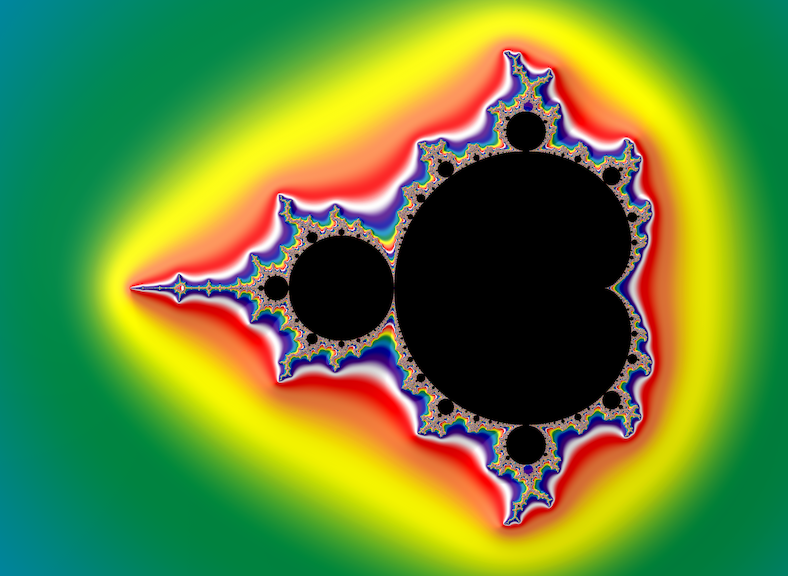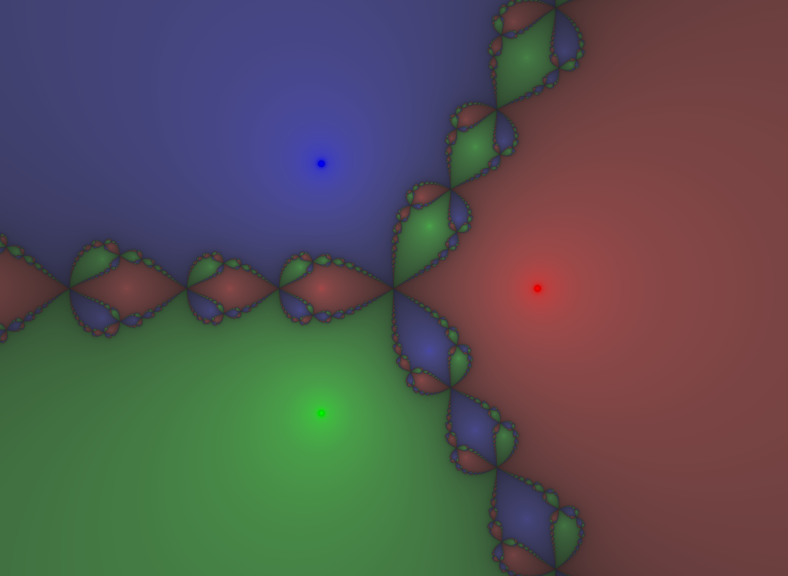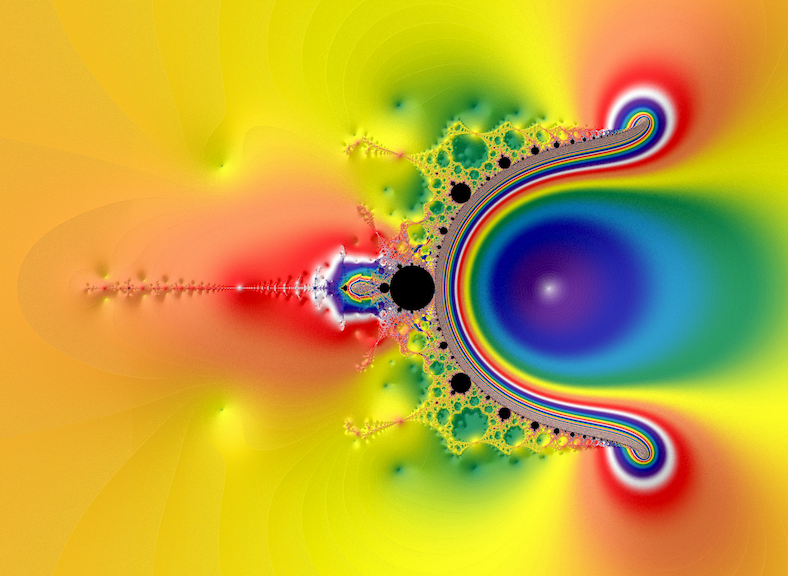# mathr / blog / #

## Perturbing NovaThe Mandelbrot set fractal is formed by iterations of $$z \to z^2 + c$$ where $$c$$ is determined by the coordinates of the pixel and $$z$$ starts at the critical point $$0$$. The critical point is where the derivative w.r.t. $$z$$ is $$0$$. The image is usually coloured by how quickly $$z$$ escapes to infinity, regions that remain bounded forever are typically coloured black. It has a distinctive shape, with a cardioid adjoined by many disks decreasing in size, each with further disks attached. Looking closely there are smaller "island" copies of the whole, but they are actually attached to the main "continent" by thin filaments.The Newton fractal is formed by applying Newton's method to the cube roots of unity, iterating $$z \to z - \frac{z^3 - 1}{3z^2}$$ where the initial $$z$$ is determined by the coordinates of the pixel. The image is usually coloured by which of the 3 complex roots of unity is reached, with brightness and saturation showing how quickly it converged. It has its own distinctive shape, with three rays extending from the origin towards infinity separating the immediate basins of attraction, each with an intricate shape: at each point on the Julia set, different points in an arbitrarily small neighbourhood will converge to all three of the roots.The Nova fractal mashes these two fractals together: the Newton fractal is perturbed with a $$+ c$$ term that is determined by the pixel, and the iteration starts from a critical point (any of the cube roots of unity). The image is coloured by how quickly the iteration converges to a fixed point (a different point for each pixel) and points that don't converge (or converge to a cycle of length greater than 1) are usually coloured black. The fractal appearance combines features of the Newton fractal and the Mandelbrot set, with mini-Mandelbrot set islands appearing in the filaments.

Deep zooms of the Mandelbrot set can be rendered efficiently using perturbation techniques: consider $z = (Z+z) - Z \to ((Z + z)^2 + (C + c)) - (Z^2 + C) = (2 Z + z) z + c$ where $$Z, C$$ is a "large" high precision reference and $$z, c$$ is a "small" low precision delta for nearby pixels. Going deeper one can notice "glitches" around mini-Mandelbrot sets when the reference is not suitable, but these can be detected with Pauldelbrot's criterion $$|Z+z| << |Z|$$, at which point you can use a different reference that might be more appropriate for the glitched pixels.

Trying to do the same thing for the Nova fractal works at first, but going deeper (to about $$10^{30}$$ zoom factor) it breaks down and glitches occur that are not fixed by using a nearer reference. These glitches are due to the non-zero critical point recurring in the periodic mini-Mandelbrot sets: precision loss occurs when mixing tiny values with large values. They also occur when affine-conjugating the quadratic Mandelbrot set to have a critical point away from zero (e.g. $$z \to z^2 - 2 z + c$$ has a critical point at $$z = 1$$). Affine-conjugation means using an affine function $$m(z) = a z + b$$ to conjugate two functions $$f, F$$ like $$m(f(z)) = F(m(z))$$.

The solution is to affine-conjugate the Nova fractal formula, to move the starting critical point from $$1$$ to $$0$$. One way of doing it gives the modified Nova formula $z \to \frac{ \frac{2}{3} z^3 - 2 z - 1 }{ (z + 1)^2 } + c + 1$ which seems to work fine when going beyond $$10^{30}$$ at the same locations where the variant with critical point $$z=1$$ fails. For example, see the ends of the following two short videos: